Next: Results of quality assessment Up: Methods of quality assessment Previous: Short Time Observables

## Long Time Observables

To estimate the influence of the applied approximations on the description of protein structure and dynamics, we have extracted the following observables from the long time simulations. As a measure for the temporal evolution of protein structure we have chosen the rms deviations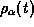of the intermediate configurations from the initial structure. If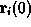are the atomic positions obtained after the equilibration phase, the rms deviations of the set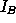of heavy backbone atoms (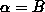) and of the corresponding set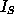of side chain atoms (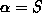) are given by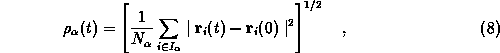where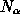denotes the number of atoms in the set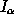.

To estimate approximation effects on protein dynamics we monitor correlations between the motions of atoms i and j by the normalized covariance matrix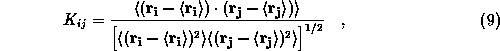where the averages are taken over trajectory sections specified further below.

Subsequently we compare covariance matrices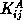and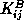from simulations A and B, respectively, both by a graphical method and by a single numerical observable. The latter is the rms difference of the cross correlations, i.e.,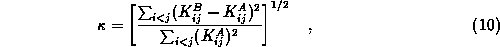whereas the former is an overlay of certain contour plots pertaining to the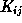. These contour plots visualize histograms representing the frequencies of values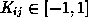as functions of atomic distances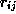and will be called covariance plots.

As a technical point we would like to note that comparisons of the type sketched above require, that the trajectories refer to one and the same conformational substate of the protein considered. The reason is, that dynamical correlations may vary from substate to substate, such that differences of these correlations, which are caused by the application of different algorithms, may be obscured.

Helmut Grubmueller
Wed Apr 30 15:40:09 MET DST 1997